What animal items should we use in the NextKids experiment? Ideally, we’d want them to (a) be sufficiently similar yet diverse enough to reveal interesting embeddings, (b) have high naming agreement, and (c) have age of acquistion (aoa) variability so we can look at embeddings as a function vocab size.

Martin, Clint, and I discussed using the Snodgrass and Vanderwart pictures (1980, n = 270), since there are already lots of exisiting norms on them. Note that one question here is whether we want to use the black and white or the (newer) colored versions, eg:

``knitr::include_graphics(c("pics/021.jpg", "pics/021_color.jpg"))``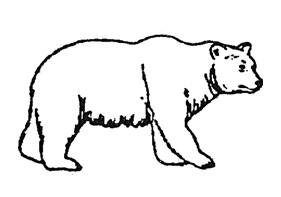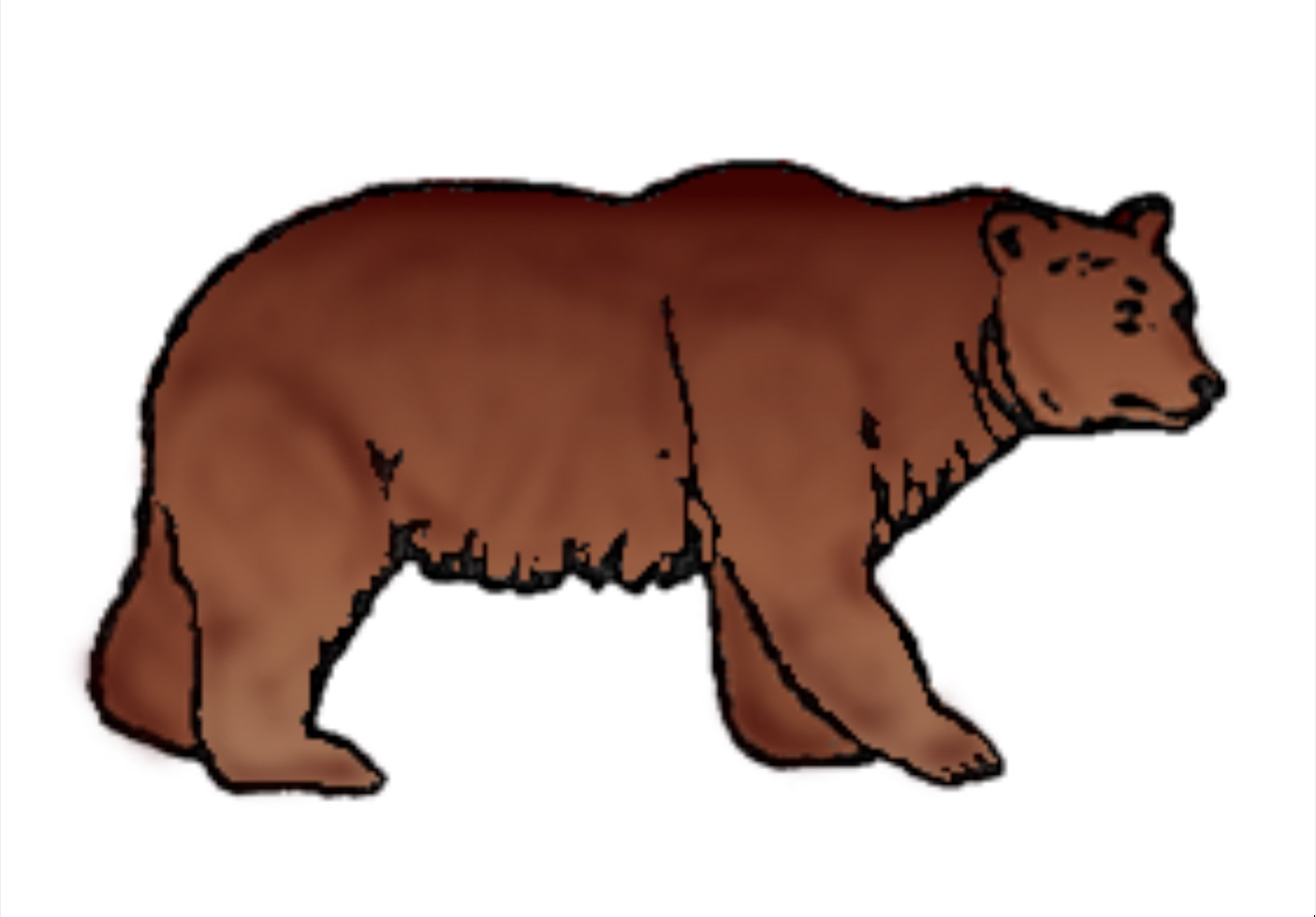``knitr::include_graphics(c("pics/003.jpg","pics/003_color.jpg"))``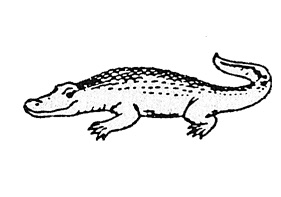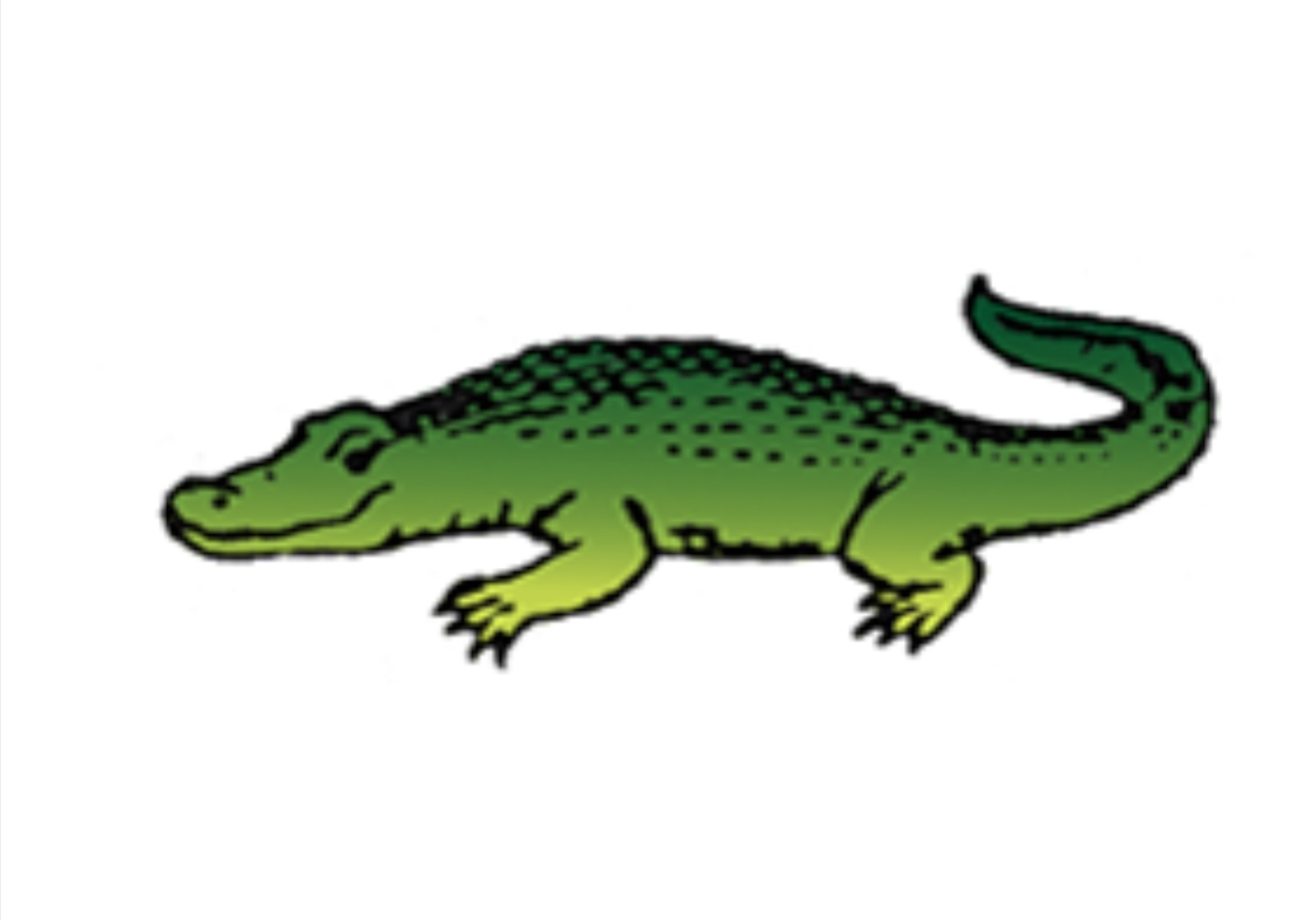There are 43 animals in the Snodgrass and Vanderwart set. The next question is which subset to use, which depends on how many items we need. In terms of similarity, I’m not totally sure how to go about selecting a set of items with the right level of diversity (Would it be useful to make use of existing similarity norms to guide our choice e.g. from this paper?).

In terms of naming agreement, Martin and Clint found this this very useful paper, which has norms for the SV pictures, as rated by 5- and 6- year olds.

In terms of aoa, I estimated aoas using the Wordbank dataset, which is based on parent report checklists (CDI). 17 of 43 of SV items are not on the CDI, so for those items I estimated aoa using adult aoa estimates, rescaled based on the wordbank data.

``````## Read in data, clean and merge.

# norms for Snodagrass and Vanderwart pictures (from Cyowicz, et al. 1997)

# wordbank aoas
rename(wb.aoa = aoa) %>%
filter(category == "animals") %>%
mutate(definition = unlist(lapply(strsplit(as.character(definition),
"\\("), function(x) x)),
definition = str_trim(definition))

# Kuperman et al AOAs (for missing values from wordbank)
select(Word, Rating.Mean) %>%

sna = snod.norms %>%
left_join(aoa.norms.wb %>% select(wb.aoa, definition),
c("Intentional.name" = "definition")) %>%
left_join(aoa.norms.kuperman, c("Intentional.name"="Word")) %>%
filter(is_insect == 0) %>%
select(-is_insect, -modal.name) %>%
arrange(wb.aoa)``````
``````## Rescale adult aoas for missing wb aoas.
scale.params = summary(lm(wb.aoa ~ adult.aoa, sna)) %>%
tidy() %>%
select(estimate)

intercept = scale.params[1,1]
slope = scale.params[2,1]

sna = sna %>%
mutate(all.aoa = ifelse(is.na(wb.aoa),
imputed = ifelse(is.na(wb.aoa), "estimated", "reported")) %>%
arrange(all.aoa) %>%
mutate(n = 1:n())

#ggplot(sna, aes(x = all.aoa, y = adult.aoa)) +
#  geom_point(aes(color = imputed), size = 2) +
#  geom_smooth(method = "lm") ``````

## Correlations between norms

Aoas are negatively correlated with percent agreement (words that are learned later have less naming agreement). Familarity and (visual) complexity are additional child-rated measures.

``````correlate(sna %>%  select(4:6,9)) %>%
shave() %>%
fashion() %>%
kable()``````
rowname percent_agreement Familiarity Complexity all.aoa
percent_agreement
Familiarity .40
Complexity -.39 -.26
all.aoa -.65 -.46 .25

## Distribution of aoas and percentage agreement

Aoas are given in months and denote the age at which at least 50 percent of children know the word. The red dash reference line in the left facet corresponds to two years of age.

``````sna %>%
gather(measure, value, c(4,9)) %>%
mutate(ref = ifelse(measure == "all.aoa", 24, 50)) %>%
ggplot(aes(x = value)) +
geom_histogram() +
geom_vline(aes(xintercept = ref), linetype = 2, color = "red") +
facet_wrap(~measure, scales = "free_x") +
theme_bw()``````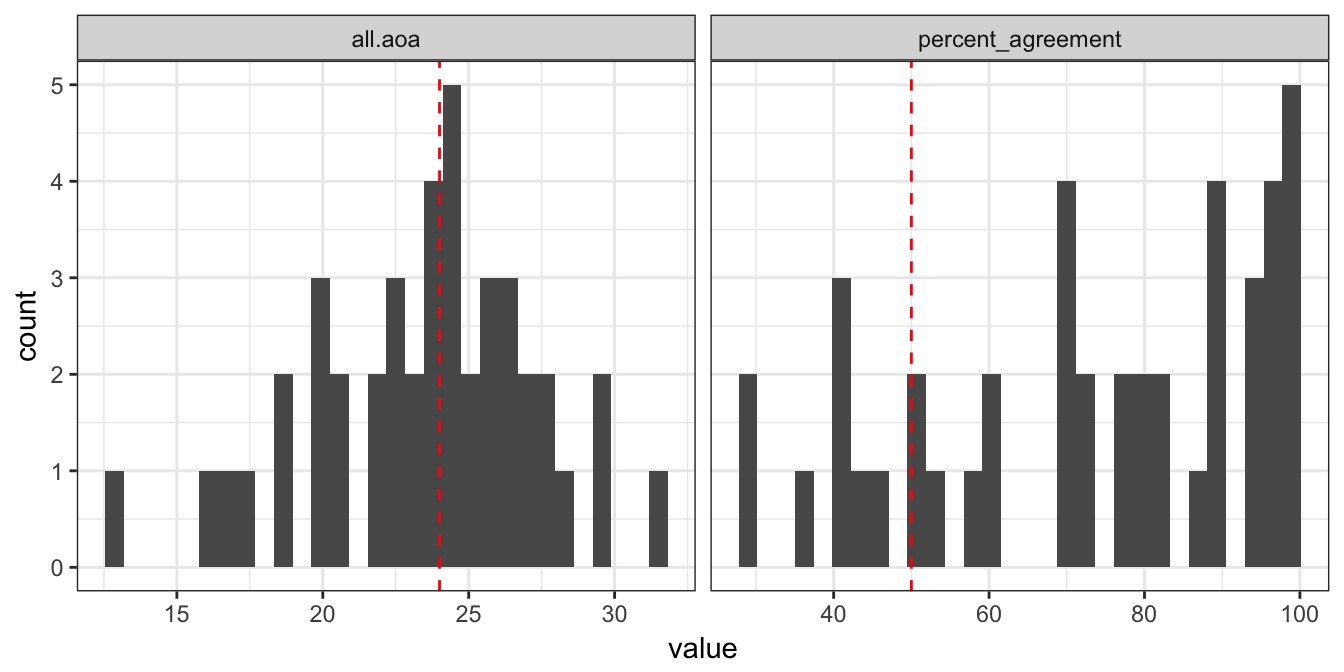## All items

(sorted by aoa)

``````kable(sna %>% select(11,2,9,4,5,6),
col.names = c("", "name", "aoa (months)", "% child agreement",
"child familiarity", "child visual complexity"))``````